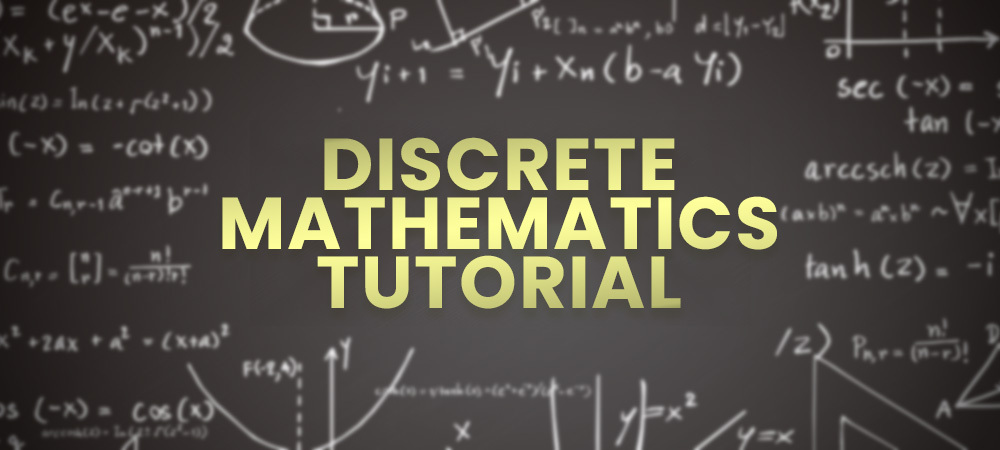# Discrete Mathematics Tutorial

Discrete Mathematics is a branch of mathematics that is concerned with “discrete” mathematical structures instead of “continuous”. Discrete mathematical structures include objects with distinct values like graphs, integers, logic-based statements, etc. In this tutorial, we have covered all the topics of Discrete Mathematics for computer science like set theory, recurrence relation, group theory, and graph theory.Recent Articles on Discrete Mathematics!